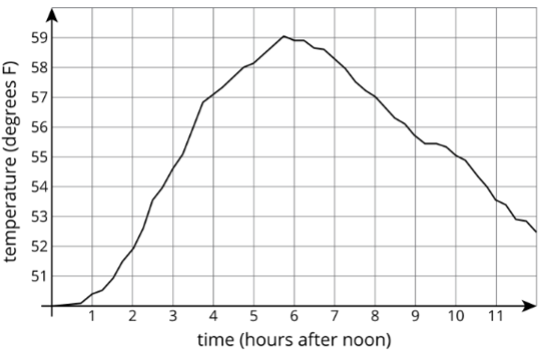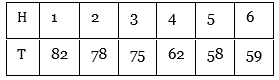Maths-
General
Easy

Question

# The graph shows the temperature between noon and midnight in City A on a certain day.The table shows the temperature, TT, in degrees Fahrenheit, for hh hours after noon, in City B.1.Which city was warmer at  p.m.?2.Which city had a bigger change in temperature between  p.m. and  p.m.?3.How much greater was the highest recorded temperature in  than the highest recorded temperature in City A during this time?4.Compare the outputs of the functions when the input is 3 .Hint:

## The correct answer is: graph and table correctly to find the correct answer.

### Step by step solution:1.From the graph, we can observe thatThe temperature in city A at 4:00 pm = 57 FFrom the table, it can be seen thatThe temperature in city B at 4:00 PM = 62 FComparing the above two values, we get that city B was warmer at 4:00PM2.For city A:Temperature at 1:00 PM = 50.5 F (approx)Temperature at 5:00 PM = 58 FChange in temperature = (58-50.5=) 8.5 FFor city B:Temperature at 1:00 PM = 82 FTemperature at 5:00 PM = 58 FChange in temperature = (58-82=) -24 FThus, there is a bigger change in temperature between 1:00 PM and 5:00 PM in city B.3.From the graph,The highest recorded temperature in City A = 59 FFrom the table,The highest recorded temperature in City B = 82 FHence, the highest recorded temperature in City B is greater than the highest recorded temperature in City A by (82-59) F = 23 F4.Assuming that the input is the time after noon, we need to find the output which is the temperature at that time.Input is given to be 3So,Temperature in city A at 3:00 PM = 54.5F (approx)Temperature in city B at 3:00 PM = 75 F

Here, we just need to read the given question carefully and understand each and every term. We need to interpret the graph and table correctly to find the correct answer.#### With Turito Foundation.#### Get an Expert Advice From Turito.